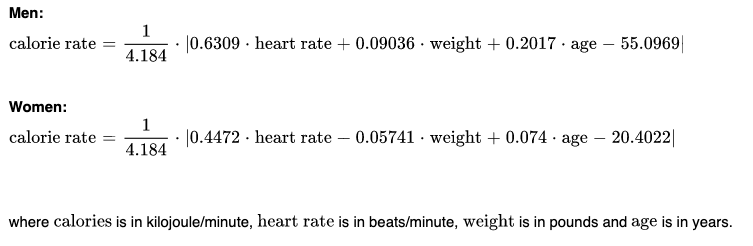# How are Calories Calculated on the ELEMNT and Wahoo Fitness App?

For the best measure of calories burned, the ELEMNT GPS Bike Computer series (including the ELEMNT, ELEMNT BOLT, and ELEMNT ROAM) and Wahoo Fitness app default to using calculations based on power output when a trainer or power meter are connected (if no direct power source is connected, heart rate will be used to calculate calories as described further below). Calories from power are calculated based on the following formula:

• Calorie rate = (4.184kJ) * 0.239GMR

In the calculation above, Kilo Joules (kJ) are measured from the trainer or power meter and multiplied by the Gross Metabolic Rate (GMR, an average of gross metabolic efficiency), estimated at 23.9% since 1 Calorie is equal to 4.184kJ of work and the human body is about 24% efficient at burning calories. In practice, this means that Calories burned will often match or closely follow power output as measured in kJ. For more info on Calorie calculations based on power, please see this SYSTM page for an in-depth discussion that matches Wahoo calculations.

While power is generally the best measure of calorie expenditure, during activity a strong relationship remains between heart rate and calories burned. The ELEMNT GPS Bike Computer series (including the ELEMNT, ELEMNT BOLT, and ELEMNT ROAM) and Wahoo Fitness app leverage this relationship to calculate the calories burned when a heart rate sensor is connected when no power source is available. The calories burned calculation uses the following equations from a study by Keytel et al. (2005)* as cited below:This equation is evaluated once per second. The results are converted to kilojoules per second, then accumulated to calculate total calories burned.

*Reference:

Keytel, L. R., Goedecke, J. H., Noakes, T. D., Hiiloskorpi, H., Laukkanen, R., van der Merwe, L., & Lambert, E. V. (2005). Prediction of energy expenditure from heart rate monitoring during submaximal exercise. Journal of Sports Sciences, 23(3), 289–297.